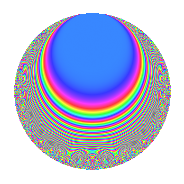# Properties

 Label 1003.2.a.jLevel 1003 Weight 2 Character orbit 1003.a Self dual Yes Analytic conductor 8.009 Analytic rank 0 Dimension 22 CM No

# Learn more about

## Newspace parameters

 Level: $$N$$ = $$1003 = 17 \cdot 59$$ Weight: $$k$$ = $$2$$ Character orbit: $$[\chi]$$ = 1003.a (trivial)

## Newform invariants

 Self dual: Yes Analytic conductor: $$8.00899532273$$ Analytic rank: $$0$$ Dimension: $$22$$ Fricke sign: $$-1$$ Sato-Tate group: $\mathrm{SU}(2)$

## $q$-expansion

The dimension is sufficiently large that we do not compute an algebraic $$q$$-expansion, but we have computed the trace expansion.

 $$\operatorname{Tr}(f)(q) =$$ $$22q$$ $$\mathstrut +\mathstrut 5q^{2}$$ $$\mathstrut +\mathstrut 7q^{3}$$ $$\mathstrut +\mathstrut 25q^{4}$$ $$\mathstrut +\mathstrut 19q^{5}$$ $$\mathstrut +\mathstrut 5q^{6}$$ $$\mathstrut +\mathstrut 3q^{7}$$ $$\mathstrut +\mathstrut 21q^{8}$$ $$\mathstrut +\mathstrut 31q^{9}$$ $$\mathstrut +\mathstrut O(q^{10})$$ $$\operatorname{Tr}(f)(q) =$$ $$22q$$ $$\mathstrut +\mathstrut 5q^{2}$$ $$\mathstrut +\mathstrut 7q^{3}$$ $$\mathstrut +\mathstrut 25q^{4}$$ $$\mathstrut +\mathstrut 19q^{5}$$ $$\mathstrut +\mathstrut 5q^{6}$$ $$\mathstrut +\mathstrut 3q^{7}$$ $$\mathstrut +\mathstrut 21q^{8}$$ $$\mathstrut +\mathstrut 31q^{9}$$ $$\mathstrut +\mathstrut 8q^{11}$$ $$\mathstrut +\mathstrut 20q^{12}$$ $$\mathstrut +\mathstrut 14q^{13}$$ $$\mathstrut +\mathstrut 5q^{14}$$ $$\mathstrut +\mathstrut 15q^{15}$$ $$\mathstrut +\mathstrut 23q^{16}$$ $$\mathstrut -\mathstrut 22q^{17}$$ $$\mathstrut +\mathstrut 6q^{18}$$ $$\mathstrut +\mathstrut 6q^{19}$$ $$\mathstrut +\mathstrut 43q^{20}$$ $$\mathstrut +\mathstrut 8q^{21}$$ $$\mathstrut -\mathstrut 8q^{22}$$ $$\mathstrut +\mathstrut 15q^{23}$$ $$\mathstrut -\mathstrut 9q^{24}$$ $$\mathstrut +\mathstrut 33q^{25}$$ $$\mathstrut +\mathstrut 9q^{26}$$ $$\mathstrut +\mathstrut 25q^{27}$$ $$\mathstrut +\mathstrut 11q^{28}$$ $$\mathstrut -\mathstrut q^{29}$$ $$\mathstrut -\mathstrut 51q^{30}$$ $$\mathstrut -\mathstrut 9q^{31}$$ $$\mathstrut +\mathstrut 37q^{32}$$ $$\mathstrut +\mathstrut 21q^{33}$$ $$\mathstrut -\mathstrut 5q^{34}$$ $$\mathstrut +\mathstrut 29q^{35}$$ $$\mathstrut +\mathstrut 30q^{36}$$ $$\mathstrut -\mathstrut 2q^{37}$$ $$\mathstrut +\mathstrut 39q^{38}$$ $$\mathstrut +\mathstrut 4q^{39}$$ $$\mathstrut +\mathstrut 4q^{40}$$ $$\mathstrut +\mathstrut 21q^{41}$$ $$\mathstrut -\mathstrut 65q^{42}$$ $$\mathstrut +\mathstrut q^{43}$$ $$\mathstrut +\mathstrut 17q^{44}$$ $$\mathstrut +\mathstrut 65q^{45}$$ $$\mathstrut -\mathstrut 39q^{46}$$ $$\mathstrut +\mathstrut 37q^{47}$$ $$\mathstrut +\mathstrut 15q^{48}$$ $$\mathstrut +\mathstrut 25q^{49}$$ $$\mathstrut -\mathstrut 48q^{50}$$ $$\mathstrut -\mathstrut 7q^{51}$$ $$\mathstrut +\mathstrut 7q^{52}$$ $$\mathstrut +\mathstrut 69q^{53}$$ $$\mathstrut +\mathstrut 13q^{54}$$ $$\mathstrut +\mathstrut 10q^{55}$$ $$\mathstrut -\mathstrut 33q^{56}$$ $$\mathstrut -\mathstrut 4q^{57}$$ $$\mathstrut +\mathstrut 4q^{58}$$ $$\mathstrut +\mathstrut 22q^{59}$$ $$\mathstrut +\mathstrut 18q^{60}$$ $$\mathstrut -\mathstrut 29q^{61}$$ $$\mathstrut +\mathstrut 29q^{62}$$ $$\mathstrut +\mathstrut 7q^{63}$$ $$\mathstrut -\mathstrut 3q^{64}$$ $$\mathstrut +\mathstrut 25q^{65}$$ $$\mathstrut -\mathstrut 16q^{66}$$ $$\mathstrut -\mathstrut 10q^{67}$$ $$\mathstrut -\mathstrut 25q^{68}$$ $$\mathstrut +\mathstrut 26q^{69}$$ $$\mathstrut +\mathstrut 29q^{70}$$ $$\mathstrut +\mathstrut 3q^{71}$$ $$\mathstrut +\mathstrut 53q^{72}$$ $$\mathstrut -\mathstrut 4q^{73}$$ $$\mathstrut +\mathstrut 13q^{74}$$ $$\mathstrut -\mathstrut 8q^{75}$$ $$\mathstrut -\mathstrut 13q^{76}$$ $$\mathstrut +\mathstrut 71q^{77}$$ $$\mathstrut +\mathstrut 11q^{78}$$ $$\mathstrut -\mathstrut 20q^{79}$$ $$\mathstrut -\mathstrut 9q^{80}$$ $$\mathstrut +\mathstrut 42q^{81}$$ $$\mathstrut +\mathstrut 11q^{82}$$ $$\mathstrut +\mathstrut 24q^{83}$$ $$\mathstrut -\mathstrut 92q^{84}$$ $$\mathstrut -\mathstrut 19q^{85}$$ $$\mathstrut -\mathstrut 10q^{86}$$ $$\mathstrut -\mathstrut 4q^{87}$$ $$\mathstrut +\mathstrut 2q^{88}$$ $$\mathstrut +\mathstrut 40q^{89}$$ $$\mathstrut -\mathstrut 78q^{90}$$ $$\mathstrut -\mathstrut 31q^{91}$$ $$\mathstrut -\mathstrut 39q^{92}$$ $$\mathstrut +\mathstrut 53q^{93}$$ $$\mathstrut +\mathstrut 32q^{94}$$ $$\mathstrut +\mathstrut 42q^{95}$$ $$\mathstrut -\mathstrut 36q^{96}$$ $$\mathstrut +\mathstrut 13q^{97}$$ $$\mathstrut -\mathstrut 15q^{98}$$ $$\mathstrut -\mathstrut 64q^{99}$$ $$\mathstrut +\mathstrut O(q^{100})$$

## Embeddings

For each embedding $$\iota_m$$ of the coefficient field, the values $$\iota_m(a_n)$$ are shown below.

For more information on an embedded modular form you can click on its label.

Label $$a_{2}$$ $$a_{3}$$ $$a_{4}$$ $$a_{5}$$ $$a_{6}$$ $$a_{7}$$ $$a_{8}$$ $$a_{9}$$ $$a_{10}$$
1.1 −2.50776 1.17790 4.28884 3.99303 −2.95389 3.24590 −5.73985 −1.61255 −10.0135
1.2 −2.28467 0.0353698 3.21973 −0.992800 −0.0808085 −0.288469 −2.78667 −2.99875 2.26822
1.3 −2.18647 3.36438 2.78064 4.25307 −7.35611 −0.847258 −1.70683 8.31907 −9.29919
1.4 −2.02992 −1.59232 2.12059 −2.38274 3.23228 −2.16746 −0.244779 −0.464532 4.83678
1.5 −1.46853 2.37414 0.156587 0.0990345 −3.48650 4.77589 2.70711 2.63653 −0.145435
1.6 −1.37758 −3.34566 −0.102265 1.06817 4.60892 2.01707 2.89604 8.19343 −1.47150
1.7 −1.25705 −2.18501 −0.419822 4.45642 2.74667 −4.53135 3.04184 1.77428 −5.60195
1.8 −0.921671 −0.437600 −1.15052 −1.68123 0.403323 −3.39099 2.90375 −2.80851 1.54954
1.9 −0.607530 2.06889 −1.63091 2.69382 −1.25692 −3.19752 2.20589 1.28032 −1.63658
1.10 −0.0191532 1.86649 −1.99963 −3.05402 −0.0357493 −1.55451 0.0766058 0.483795 0.0584943
1.11 0.296053 −0.947860 −1.91235 1.42120 −0.280617 3.81838 −1.15826 −2.10156 0.420750
1.12 0.324383 2.83368 −1.89478 2.69264 0.919198 3.71446 −1.26340 5.02975 0.873446
1.13 0.369502 −1.38817 −1.86347 −1.75953 −0.512933 1.96987 −1.42756 −1.07297 −0.650150
1.14 1.00923 −2.57166 −0.981445 −2.22902 −2.59541 −3.29508 −3.00898 3.61346 −2.24961
1.15 1.49676 −1.13573 0.240278 3.48120 −1.69992 −1.05586 −2.63387 −1.71011 5.21050
1.16 1.71910 3.15409 0.955303 −1.04866 5.42219 1.98044 −1.79594 6.94828 −1.80276
1.17 2.05162 1.73406 2.20914 2.98180 3.55764 −0.740180 0.429083 0.00697358 6.11752
1.18 2.12841 2.12034 2.53015 1.18258 4.51297 0.555884 1.12837 1.49586 2.51702
1.19 2.29289 −0.141514 3.25733 2.10475 −0.324476 3.17762 2.88291 −2.97997 4.82596
1.20 2.59194 −2.74602 4.71816 3.63003 −7.11751 3.18482 7.04532 4.54060 9.40883
See all 22 embeddings
 $$n$$: e.g. 2-40 or 990-1000 Embeddings: e.g. 1-3 or 1.22 Significant digits: Format: Complex embeddings Normalized embeddings Satake parameters Satake angles

## Inner twists

This newform does not have CM; other inner twists have not been computed.

## Atkin-Lehner signs

$$p$$ Sign
$$17$$ $$1$$
$$59$$ $$-1$$

## Hecke kernels

This newform can be constructed as the intersection of the kernels of the following linear operators acting on $$S_{2}^{\mathrm{new}}(\Gamma_0(1003))$$:

 $$T_{2}^{22} - \cdots$$ $$T_{3}^{22} - \cdots$$